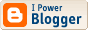## Wolfram|Alpha: Knowledge-Based Computing

WolframAlpha is a new online initiative from the company who created Mathematica. As the blog states it is not just a place to look up facts. Under the covers it uses Mathematica's inbuilt algorithms:
For example, if you give WolframAlpha a mathematical formula, a polynomial say, or something involving sines and cosines, it will give you back a number of useful results: a graph of the function, a list of its zeros, factored and expanded forms, etc.

Try this one: integral of e^(-t^2) dt, t=-infinity to infinity

<< Home###### Contact

MSN, Email: mitch døt wheat at gmail.com